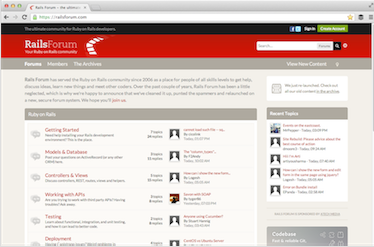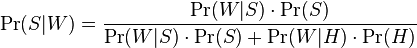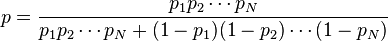aTech recently were responsible for the complete re-launch of Rails Forum - a message board for Ruby on Rails developers. Unfortunately, the previous incarnation had filled with spam due to neglect and we needed to get rid of months of spam as quickly as possible in order to archive the old content without the spam. We're pleased to say the new site, with a lovely new design has been a success with the members and we're looking forward to see how it grows again over the next few years.What follows is a post by Alex Coplan, a sixth form student from Bryanston School who has been with us this week. Alex was asked to go through the old forum and remove the spam and, not fancying doing this by hand, did so by writing some scripts to analyse the database. This is his story...

Everyone knows how to prevent spam - you ask the bots a question they won't be very good at. If the odd clever bot or human spammer slips through you can just delete them and ban their IP, no problem. But what happens if you leave spam unmoderated? This is what happened to the old railsforum.com, aside from there being a lack of effective spam prevention in the first place, the spam was left unmoderated and it became overrun with spammers. The question is, how do you get rid of thousands of spam posts when the situation is beyond the powers of manual moderation?

## First thoughts

Initially, you might think you can spot a pattern in the spam. Maybe you could write a few clever queries that would match some patterns in the spam? This works for the most blatant spam that you might find. Unfortunately, most spam does not exhibit obviously searchable patterns. The first approach I tried was to look at the spam and write a list of obviously spammy words, and then rank the posts based on the number of matches of these words found in each post. This had some success, but there are some major problems with this method. Firstly, it gives you a statistically meaningless value - a score of how many hits each post had. This does allow you to rank the posts and then look at the spammiest posts, but since it is not a probability, you can't reliably make decisions on it. The second problem is the high false positive rate. Because the "spammy" words are manually identified, you can often find words that in the context of spam appear to be a good indicator of spam, but in other contexts that you perhaps hadn't considered are perfectly valid and do not indicate spam at all. Another problem with this method is that there will always be things that you miss when identifying keywords and therefore a large quantity of the spam will be undetected.

What you need in a method is something that will thoroughly analyse all the data in each post and then return a probability that the post is spam. You also want this system to have a low false-positive rate and to be adaptable to new types of spam.

## Something a bit more fancy

After a bit of research, I decided to implement a Bayesian classifier to analyse the posts. The idea here is that we split posts into tokens, classify a training set of posts as either spam or ham, and build a database of tokens and the frequency at which they occur in both spam and ham posts. The tokeniser is relatively simple - we only consider alphanumeric characters, hyphens, apostrophes, exclamation marks and pound and dollar symbols to be part of tokens. We also ignore any tokens that consist solely of numbers, as they are relatively useless in classifying spam. Such a tokeniser might look like this:

``````def tokenise(str)
# build charset
a = ('A'..'Z').to_a
c = a + a.map(&:downcase)
c += ('0'..'9').to_a
c += ["!","-","'","\$","Â£"]

tokens = []
buff = ""
str.each_char do |ch|
if c.include? ch
buff << ch
elsif buff.length > 0
tokens << buff unless buff =~ /\A\d+\Z/
buff = ""
end
end

tokens << buff if buff.length > 0 and not buff =~ /\A\d+\Z/
tokens
end
``````

Once the posts can be tokenised, we can then start to train the classifier. I started off by getting my script to select a batch of 20 or so of the most recent posts from the forum database and present them one by one, prompting for a yes or no response as to whether the post was spam. Once we have classified a post from the training set, it can be tokenised, and I store the tokens locally in an SQLite database. As well as keeping track of the number of times each token occurs in either spam or ham posts, it is also important to keep track of the total number of posts that have been reviewed as either spam or ham. This is used later in calculating probabilities.

Once I had trained the system on a small sample of ~100 posts, I started to get it to generate a Bayesian probability on each post to see how accurately it was predicting spam posts. This probability is eventually what we're going to use to detect and remove spam users and their posts.

In order to generate a spam probability (or "spamicity") of a post, the spamicity of each token in the post is calculated and the probabilities for each token are combined.

Wikipedia gives the formula for determining the spamicity of a given word (based on Bayes' theorem) as follows:This formula describes the spamicity of a word (probability that a message is spam given the occurrence of the word W). P(W|S) is the probability of the word W appearing in spam, P(S) is the probability of a spam message being chosen randomly from the sample, P(W|H) is the probability of W occurring in a ham message, and P(H) is the probability of a ham message being chosen randomly from our training sample.

In practice, the code for this might look like so:

``````total_corpus = spam_count + ham_count
pr_spam = spam_count / total_corpus
pr_ham = ham_count / total_corpus

pr_word_given_spam = spam_occurrences / spam_count
pr_word_given_ham = ham_occurrences / ham_count
spamicity_word = pr_word_given_spam * pr_spam / (pr_word_given_spam * pr_spam + pr_word_given_ham * pr_ham)
spamicity_word = 0.998 if spamicity_word == 1
spamicity_word = 0.001 if spamicity_word == 0
``````

Here I refer to the corpus as the total body of posts that have been reviewed so far. `spam_count` and `ham_count` are the total number of spam and ham message in the corpus. `spam_occurrences` and `ham_occurrences` are the number of occurrences of our given word in either spam or ham. We also make an assumption here that if a word has only ever been seen by our classifier as spam (thus spamicity of 1) or as ham (spamicity of 0), then we change the probability to be 0.998 or 0.001 respectively, as otherwise it would override the other probabilities when they were combined.

Looping through each token in a post, I assign the spamicity of each token to a hash, using the token as a key. From this hash we can then get 15 tokens with the most significant (extreme) probabilities. This is an essential part of the classifier, as by using the extreme (i.e. very spammy or very hammy) probabilities then only the tokens which are actually useful in classifying the post are taken into account. This makes the resultant probability much more clear cut (i.e. very close to 0 or very close to 1) and also means that neutral words (words that appear equally frequently in both spam and ham) do not affect the final probability. This is done like so, by calculating the distance of each value from 0.5:

``````significant = tokens.sort_by { |k, v| (0.5-v).abs }.last(15) # top 15 most significant tokens
``````

We then need to combine the probabilities for these significant tokens, using another formula derived from Bayes' theorem:This formula simply says to divide the product of the probabilities by the sum of the product of the probabilities and the product of the inverse (1-p) probabilities. We can combine them in code like so:

``````spamicities = significant.map { |a| a } # get just the probabilities in an array
product = spamicities.inject(&:*)
inverse_product = spamicities.map { |s| 1-s  }.inject(&:*)
probability = product / (product + inverse_product)
``````

Another important point to consider now is how we deal with tokens that the classifier previously hasn't seen before (and therefore has no probability for). A useful way of dealing with this problem is to degrade more complex tokens into simpler tokens. For example, matching missing tokens that are in upper or mixed case can be done by matching such tokens in lowercase. Another method is to remove trailing characters such as exclamation marks or other non-alphanumeric accepted token characters. A method such as the following can generate a simpler token to match if an exact token match is not found:

``````def make_simple_token(token)
t = token.downcase
new_t = t
(t.length-1).downto(1) do |i|
ch = t[i]
if ["!","-","'","\$","Â£"].include?(ch)
new_t = new_t[0..-2]
else
return new_t
end
end
new_t
end
``````

If neither the original or simple token can be matched, then we must make an assumption about the probability. In order to guard against false-positives, we slightly bias this probability to ham by choosing 0.4. This value (along with many of my other assumptions) is used by Paul Graham in the spam filter described in his article A Plan for Spam.

Finally we can start to see that our training is having an effect. By getting the training script to calculate the spamicity of our post before getting us to rate it, we can get a good idea of how well it is doing, and how much more training might be needed. I ended up training my classifier on ~2000 posts from the forum. However, I was using it to detect spam users with a training sample of only ~1000 posts.

Now that we have a trained classifier comes the fun part; destroying the spam users and all their posts. The way I did this was to select a batch of say 1000 of the most recently registered users. For each user my script analysed their posts, generating a Bayesian spam probability for each of them. If the maximum probability for that user's posts was greater than 50%, then I generated a summary of that user. This included their maximum and average spamicities, list of topic titles, and the full content of three of their posts with the highest spamicities. I then had to review that user, but from this information it was almost always obvious whether the user is a spammer or not. If I mark the user as spam, the system re-marks all their posts as spam, in order to improve the classifier. It then deletes all their posts, topics and finally their account. If however the classifier got this wrong (which happened surprisingly occasionally), it marks all their content as ham, and adds their username to a whitelist so that they won't be re-analysed. This method of analysis allowed me to get through the first 7000 users in a few hours, deleting all of their spam posts.

This method, from the first line of code to the last database query took about two days to implement, and removed thousands of spam posts from the forum. With a forum of around 150,000 posts, using a sample of only 1.3%, the classifier learnt to recognise almost all the spam on the forum. After having a poke around on archive.railsforum.com, I now can't find any obvious spam remaining, but do let me know if you manage to find any!